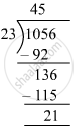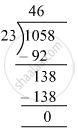# What least number must be added to 1056 to get a number exactly divisible by 23? - Mathematics

Fill in the Blanks

What least number must be added to 1056 to get a number exactly divisible by 23?

#### Solution

First, we will divide 1056 by 23.Required number = 23 − 21 = 2
So, 2 must be added to 1056 to make it exactly divisible by 23.
i.e., 1056 + 2 = 1058
Now, we have:∴ 1058 is exactly divisible by 23.

Is there an error in this question or solution?

#### APPEARS IN

RS Aggarwal Class 6 Mathematics
Chapter 3 Whole Numbers
Exercise 3E | Q 9 | Page 56